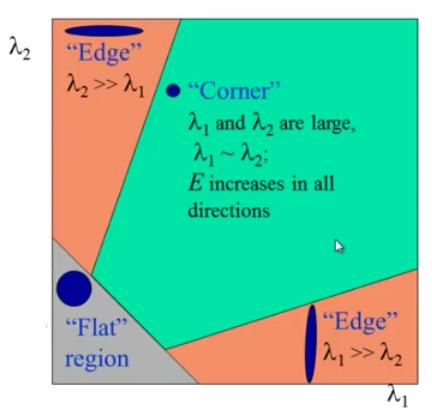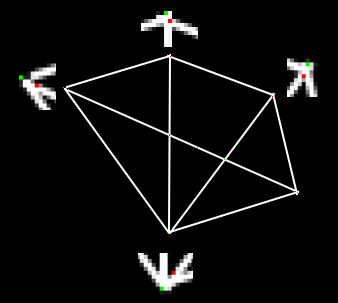# 哈里斯角检测

### 理论

$$E(u,v) = \sum_{x,y} \underbrace{w(x,y)}\text{window function} \, [\underbrace{I(x+u,y+v)}\text{shifted intensity}-\underbrace{I(x,y)}_\text{intensity}]^2$$

$$E(u,v) \approx \begin{bmatrix} u & v \end{bmatrix} M \begin{bmatrix} u \ v \end{bmatrix}$$

$$M = \sum_{x,y} w(x,y) \begin{bmatrix}I_x I_x & I_x I_y \ I_x I_y & I_y I_y \end{bmatrix}$$

$$R = det(M) - k(trace(M))^2$$

• $det(M)=\lambda_1\lambda_2$
• $trace(M)=\lambda_1+\lambda_2$
• $\lambda_1$ and $\lambda_2$ 是 $M$ 的特征值

• 当$|R|$较小，这在$\lambda_1$和$\lambda_2$较小时发生，该区域平坦。
• 当$R<0$时（当$\lambda_1 >>\lambda_2$时发生，反之亦然），该区域为边。
• 当$R$很大时，这发生在$\lambda_1$和$\lambda_2$大且$\lambda_1$~$\lambda_2$时，该区域是角。### OpenCV中的哈里斯角检测

import numpy as np
import cv2 as cv
filename = 'chessboard.png'
gray = cv.cvtColor(img,cv.COLOR_BGR2GRAY)
gray = np.float32(gray)
dst = cv.cornerHarris(gray,2,3,0.04)
#result用于标记角点，并不重要
dst = cv.dilate(dst,None)
#最佳值的阈值，它可能因图像而异。
img[dst>0.01*dst.max()]=[0,0,255]
cv.imshow('dst',img)
if cv.waitKey(0) & 0xff == 27:
cv.destroyAllWindows()### SubPixel精度的转角

import numpy as np
import cv2 as cv
filename = 'chessboard2.jpg'
gray = cv.cvtColor(img,cv.COLOR_BGR2GRAY)
# 寻找哈里斯角
gray = np.float32(gray)
dst = cv.cornerHarris(gray,2,3,0.04)
dst = cv.dilate(dst,None)
ret, dst = cv.threshold(dst,0.01*dst.max(),255,0)
dst = np.uint8(dst)
# 寻找质心
ret, labels, stats, centroids = cv.connectedComponentsWithStats(dst)
# 定义停止和完善拐角的条件
criteria = (cv.TERM_CRITERIA_EPS + cv.TERM_CRITERIA_MAX_ITER, 100, 0.001)
corners = cv.cornerSubPix(gray,np.float32(centroids),(5,5),(-1,-1),criteria)
# 绘制
res = np.hstack((centroids,corners))
res = np.int0(res)
img[res[:,1],res[:,0]]=[0,0,255]
img[res[:,3],res[:,2]] = [0,255,0]
cv.imwrite('subpixel5.png',img)